# How to Determine Sample Size, Determining Sample Size

By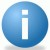iSixSigma-Editorial

In order to prove that a process has been improved, you must measure the process capability before and after improvements are implemented. This allows you to quantify the process improvement (e.g., defect reduction or productivity increase) and translate the effects into an estimated financial result – something business leaders can understand and appreciate. If data is not readily available for the process, how many members of the population should be selected to ensure that the population is properly represented? If data has been collected, how do you determine if you have enough data?

Determining sample size is a very important issue because samples that are too large may waste time, resources and money, while samples that are too small may lead to inaccurate results. In many cases, we can easily determine the minimum sample size needed to estimate a process parameter, such as the population mean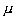.

When sample data is collected and the sample mean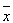is calculated, that sample mean is typically different from the population mean. This difference between the sample and population means can be thought of as an error. The margin of error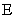is the maximum difference between the observed sample meanand the true value of the population mean:

Handpicked Content:   Use Cases and Measures: Strengthening the Six Sigma Link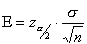where: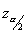is known as the critical value, the positive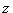value that is at the vertical boundary for the area of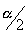in the right tail of the standard normal distribution.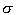is the population standard deviation.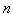is the sample size.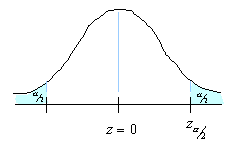Rearranging this formula, we can solve for the sample size necessary to produce results accurate to a specified confidence and margin of error.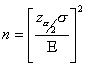This formula can be used when you knowand want to determine the sample size necessary to establish, with a confidence of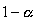, the mean valueto within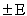. You can still use this formula if you don’t know your population standard deviationand you have a small sample size. Although it’s unlikely that you knowwhen the population mean is not known, you may be able to determinefrom a similar process or from a pilot test/simulation.

Let’s put all this statistical mumbo-jumbo to work. Take for example that we would like to start an Internet service provider (ISP) and need to estimate the average Internet usage of households in one week for our business plan and model.

### Sample Size Calculation Example

Problem
We would like to start an ISP and need to estimate the average Internet usage of households in one week for our business plan and model. How many households must we randomly select to be 95 percent sure that the sample mean is within 1 minute of the population mean. Assume that a previous survey of household usage has shown= 6.95 minutes.

Solution
We are solving for the sample size.

A 95% degree confidence corresponds to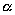= 0.05. Each of the shaded tails in the following figure has an area of= 0.025. The region to the left ofand to the right of= 0 is 0.5 – 0.025, or 0.475. In the table of the standard normal () distribution, an area of 0.475 corresponds to avalue of 1.96. The critical value is therefore= 1.96.The margin of error= 1 and the standard deviation= 6.95. Using the formula for sample size, we can calculate: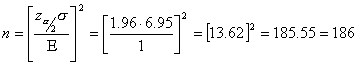So we will need to sample at least 186 (rounded up) randomly selected households. With this sample we will be 95 percent confident that the sample meanwill be within 1 minute of the true population of Internet usage.This formula can be used when you knowand want to determine the sample size necessary to establish, with a confidence of, the mean valueto within. You can still use this formula if you don’t know your population standard deviationand you have a small sample size. Although it is unlikely that you knowwhen the population mean is not known, you may be able to determinefrom a similar process or from a pilot test/simulation.
##### iSixSigma-Editorial

1.Kevin Clay

Excellent example using the startup of an Internet Service Provider (ISP)! I have moved the module of “How to Determine Sample Size” into my Six Sigma Green Belt for Service Course and have been looking for some varied well written examples. Your example fits the bill. I am going to point my students towards this article as a resource.

I look forward to reading more articles.

2.Paul

This is quite easy to understand. But can this formular be used for a two-tailed hypothesis as well?

3.S. P. Vasekar

I am electrical engineer involved in testing of relays and ehv equipments. During last 6 months some where i came across the word ‘Confidance Interval’. I have tried a lot by searching the web to get in undestood. finaly today came across this web page and got the idea of confidance interval. Also this also may relate to Cetral Limit Therom. Thanks again.

4.Arvind

Good enough. But what happens when the population is 100 or 150 ( or less than 186 for that matter).
The formula does not cover finite population. Thus 186 sample size arrived at ,should be corrected /adjusted for finite population. If the population is N, then the corrected sample size should be = (186N)/( N+185).
Example : If N=100, then the corrected sample size would be =18600/285 (=65.26 or 66)

5.Gopalakrishnan Manikandan

Hello Arvind,

Thanks for sharing this info…

6.Abdulahi Halake

I am Msc student this has help me conceptualize use of normal curve in determining sample size Thanks a lot

7.Syed Noorul hussain

You have provided good calculators

8.Pat

You mentioned twice that this formula can be used even when we do not know the population standard deviation … but you giving no example of that situation and how it would be done without knowing the standard deviation.

Also … there being another formula for sample size which using proportions (p-hat) and (1 – p-hat). How does that formula relate and compare to this formula ?

Thanks!

GOD Bless.

9.nasir

thank you . useful information.

10.manoj

i love your article. this is the only site giving a very good insights on how to calculate n.

11.Rip Stauffer

Good stuff on sample size, but you shouldn’t need any test of hypothesis to show that your project has improved a process…a pre-requisite for a capability study (before or after) is statistical process control. Comparing the control charts from the “before” process to the charts from the “after” process will show you whether you have signifcantly improved the process. If you have tracked the project metric of interest from beginning to end, you will be able to see whenever any of your “quick wins” or experiments have had an effect in near-real-time.

12.Alloch William Akoll

This explanation is very good for new students of research. Explanations are clear and illustrations are guiding. Good job done. I have personally benefited form this posting.

13.Larry D.

How does the idea of sampling and\ sample size fit into the concept of sampling from a population that has Six Sigma quality?

With a population with less than 10,000 defects in a population of one million (less than 1% defects), will sampling be effective?

I believe most of the sampling size estimating formulas were developed with the idea that the number of defects were greater than 1% of the population. That is 3.9 Six Sigma level of quality.

14.Murali N

Good one. Clearly explains the concept

15.Veronica

A city records a population of 23,000 in 2006
The statistical agency projects that by 2011, the city will hit a population of 34,000
1. How can we calculate what the population may have been in 2007, 2008, 2009, and 2010
2. How can we calculate the percentage of increase in each of these years?
3. How can we estimate the population in 2012, 2013, 2014, 2015 and 2016?
NB: I do not know the model or linear algorithm used by the statistical agency to arrive at that projection. I am simply interested in estimating or maybe I should say extrapolating what the population should be in 2016 to enable me build up some assumptions for a sample survey frame. I am carrying out a social research in the field

I just need some ideas on how to do this statistically.

I just want a general idea on how to calculate the percentage increases ( and what this might translate to in actual figures) per year.

So i can use this to do an extrapolation of some sort into 2016. I want to use this to have a rough estimate of what the population may be this year and then find a mean population
I just need some ideas on how to do this statistically

Thank you

16.Great!

17.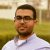Good article, What if I know only the population size (!000 for example) and I don’t know the Sigma Value ?
How can I calculate the sample size ?

18.Toivo

How do I get the sample size used if I know the (standard deviation 15), A 95% confidence interval for a population mean was reported to be 152 to 160.

19.AJ

So, if my population is 2,000,000 or even 10,000,000, that doesn’t factor into the required sample size? I can estimate the mean +/- 1 minute for a population of 10,000,000 with a sample of 186?… That just doesn’t seem right.

20.